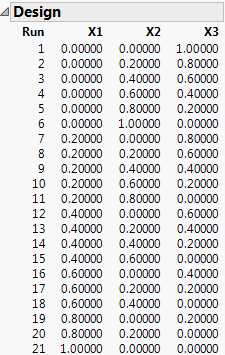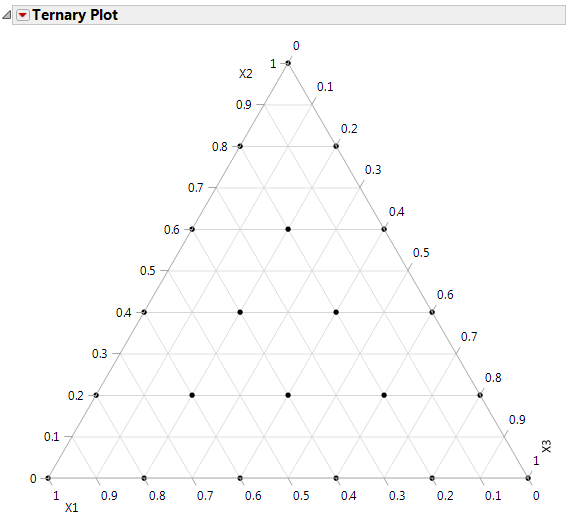Publication date: 07/30/2020

## Simplex Lattice Design

The simplex lattice design is a space filling design that creates a triangular grid of runs. The design is the set of blends where the factors’ values are i/m, where i varies from 0 to m and the sum of the factors is 1. For a three-level design, a factor can be set at 0, 0.33, 0.66, or 1.

To create an example simplex lattice design:

1. Select DOE > Classical > Mixture Design.

2. Use three factors for this example. No changes need to be made to the Factors section.

3. Click Continue.

4. Click Simplex Lattice to generate the design.

Note: The default setting of 5 levels was used. There is a text box that enables you to adjust the number of levels

Figure 13.10 Three Factor Five-Level Simplex Lattice Design5. Click Make Table.

#### Visualize the Design

6. From the design table, select Graph >Ternary Plot.

7. Select X1, X2, and X3 and click X, Plotting, and then click OK.

Figure 13.11 Ternary Plot for Simplex Lattice Design